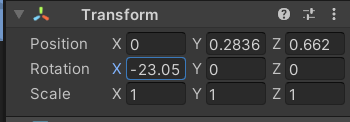# How to limit rotation of an object when pressing a key?

Currently, my gameObject is rotated up and down by pressing a key. This is my code:

``````switch (cannon){
case 0: break;
//press W
case 1:  Cannon.transform.localEulerAngles -= new Vector3(cannonRotateSpeed * Time.deltaTime, 0, 0);
// print(Cannon.transform.rotation);
;break;
//press S
case 2: Cannon.transform.localEulerAngles += new Vector3(cannonRotateSpeed * Time.deltaTime, 0, 0);
;break;
}
``````

I want when the user keeps pressing W, the Cannon rotate up but Rotation X in the inspectator capped at -25. And capped at 25 when pressing S(rotate down).You just need to get the current rotation and do a IF STATEMENT to check if it is acceptable to rotate more.

``````   switch (cannon)
{
case 0:
{
break;
}
//press W
case 1:
{
if (Cannon.transform.rotation.x > -25f)
{
Cannon.transform.localEulerAngles -= new Vector3(cannonRotateSpeed * Time.deltaTime, 0, 0);
// print(Cannon.transform.rotation);
}
break;
}
//press S
case 2:
{
if (Cannon.transform.rotation.x < 25f)
{
Cannon.transform.localEulerAngles += new Vector3(cannonRotateSpeed * Time.deltaTime, 0, 0);
}
break;
}
}
``````

There is a very simple way to clamp the rotation of the Gameobject. You need to use Mathf.Clamp01 in your situation. The above-provided solution by Knoblez is not precise and has a chance of failure due to FPS issues.

Mathf.Clamp01 is used to limit a value between two numbers.

``````        //This will clamp the rotation of the object between minRotation and maxRotation.
float minRotation = 1f;
float maxRotation = 90f;
Vector3 currentRotation = transform.localRotation.eulerAngles;
currentRotation.z = Mathf.Clamp01(currentRotation.z, minRotation, maxRotation);
transform.localRotation = Quaternion.Euler(currentRotation);
``````

Simply add this piece of code after you change the rotation of the object.